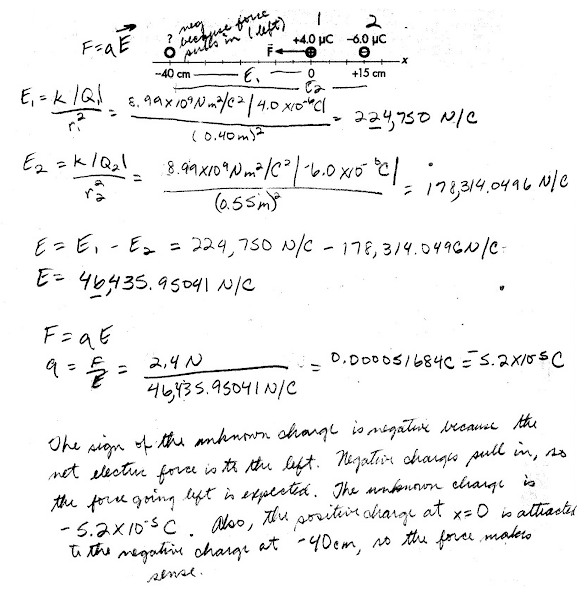## 20140329

### Physics midterm problem: known and unknown source charges exerting forces on test charge

Physics 205B Midterm 1, spring semester 2014
Cuesta College, San Luis Obispo, CA

Cf. Giambattista/Richardson/Richardson, Physics, 2/e, Problem 16.15Two point charges are held at fixed locations. An unknown charge is at  x = –40 cm, and a –6.0 µC charge is at x = +15 cm. A +4.0 µC test charge placed at the origin experiences a force of 2.4 N, directed to the left. Determine the sign (±) and amount of the unknown charge (in either coulombs or µC) that is located at x = –40 cm. Explain your reasoning using properties of electric forces, fields, and vector superposition.

• p:
Correct. Identifies (a) two source charges (Q1 = unknown charge at x = –40 cm; and Q2 = –6.0 µC charge at x = +15 cm) that exert forces on the test charge q = +4.0 µC at the origin. Then determines that since the net force on the q = +4.0 µC test charge is to the left: (b) the Q2 = –6.0 µC source charge attracts (pulls to the right) the q = +4.0 µC test charge, such that the unknown source charge Q1 must be negative in order to attract (pull to the left) the q = +4.0 µC test charge; and (c) finds the magnitude of the unknown source charge Q1 by setting up the superposition of the two force vectors on the q = +4.0 µC test charge, which is the difference of the magnitudes (due to the forces being in opposite directions), setting this equal to the total force on the test charge F = 2.4 N, and then solves for the unknown source charge Q1, which is –53 µC. May have slight but very minor conversion, arithmetic, and/or misplaced numerical values that do not substantively affect the conceptual understanding of setting up the proper vector addition.
• r:
Nearly correct, but misidentifies source charges and test charge. As (p), but identifies the unknown charge at x = –40 cm as the test charge q, having a force of 2.4 N exerted on it (either to the left or right); and the two source charges exerting forces on the unknown test charge q are Q1 = +4.0 µC at the origin, and Q2 = –6.0 µC at x = +15 cm. (In this case the numerical result for the amount of unknown test charge q = |52 µC| is coincidentally very similar to the different situation as originally described in the statement of the problem, and as executed as described in (p).)
• t:
Nearly correct, but approach has conceptual errors, and/or major/compounded math errors. At least has some attempt at identifying and executing the vector addition of two sources charges exerting forces acting on (or creating electric fields acting at the location of) a test charge.
• v:
Implementation of right ideas, but in an inconsistent, incomplete, or unorganized manner. Typically only evaluates one of the force vectors on the charge, or one of the electric field vectors at that location to determine the unknown charge.
• x:
Implementation of ideas, but credit given for effort rather than merit. Some constructive attempt at finding the force or electric field to determine the unknown charge.
• y:
Irrelevant discussion/effectively blank.
• z:
Blank.
Sections 30882, 30883
Exam code: midterm01b0w7
p: 9 students
r: 13 students
t: 9 students
v: 10 students
x: 0 students
y: 0 students
z: 0 students

A sample "p" response (from student 1391), using a direct force approach:Another sample "p" response (from student 7979), instead using a two-step electric field approach:A sample "r" response (from student 3420), using a direct force approach with the unknown charge at x = -40 cm being the test instead of a source charge:Another sample "r" response (from student 0001), instead using a two-step electric field approach with the unknown charge at x = -40 cm being the test instead of a source charge: